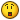## Recommended Posts

hm, how exactly works the if condition with many ands and ors... f.e.:

```If _IsPressed("26") Then
If \$a <> 0 Or \$b <> 0 Or \$c <> 0 And \$d <> 0 And \$e <> 0 Then
...
EndIf
EndIf```

is not the same as

```If _IsPressed("26") And \$a <> 0 Or \$b <> 0 Or \$c <> 0 And \$d <> 0 And \$e <> 0 Then
...
EndIf```

i jsut dont get why o.O... if you take the first example the script first get asked if the key is pressed and if it's pressed it jumps to the other if case... that is for me exactly like the 2nd code?!

in both codes it should need the key to be pressed and \$a, \$d and \$e not to be 0.

##### Share on other sites

hm, how exactly works the if condition with many ands and ors... f.e.:

```If _IsPressed("26") Then
If \$a <> 0 Or \$b <> 0 Or \$c <> 0 And \$d <> 0 And \$e <> 0 Then
...
EndIf
EndIf```

is not the same as

```If _IsPressed("26") And \$a <> 0 Or \$b <> 0 Or \$c <> 0 And \$d <> 0 And \$e <> 0 Then
...
EndIf```

i jsut dont get why o.O... if you take the first example the script first get asked if the key is pressed and if it's pressed it jumps to the other if case... that is for me exactly like the 2nd code?!

in both codes it should need the key to be pressed and \$a, \$d and \$e not to be 0.

In the second case if the key pressed is "26" and \$a = 0 then testing stops because we have a false. In the second case if the key pressed is "26" and \$a = 0 testing continues. The reason is because and and or have the same precedence so testing proceeds from left to right until a conclusion. I think you can make these these the same by adding brackets -- And (\$a <> 0 .... \$e <> 0)

##### Share on other sites

ahhh, thx... i was very confused about it, but but your solution sounds logically ^^

Edited by Lord_Doominik

##### Share on other sites

ahhh, thx... i was very confused about it, but but your solution sounds logically ^^

It does "sound" logical, but it is not logically sound - sorry. Let me give a proper example as to why these two expressions will give a different result.

In the first case to get a true result you have to have key pressed = "26" and then if that is true you need one of \$a,\$b,\$c to be <> 0 together with both \$d <> 0 and \$e <> 0.

In the second case there is a possibility of a true when the key pressed is not 26

For example if

\$b <> 0, \$d <> 0, \$e <> 0

then you get a true.

This is a direct result of left to right calculating and it seems that if you are going to use a mixture of and's and or' in an expression it is always safer to put brackets in to ensure that you get the result you want.

##### Share on other sites

yes, i said your SOLUTION sounds logically... read more carefully, i didn't said that my problem sounds logically## Create an account

Register a new account

• ### Recently Browsing   0 members

×

• Wiki

• Back

• #### Beta

• Git
• FAQ
• Our Picks
×
• Create New...